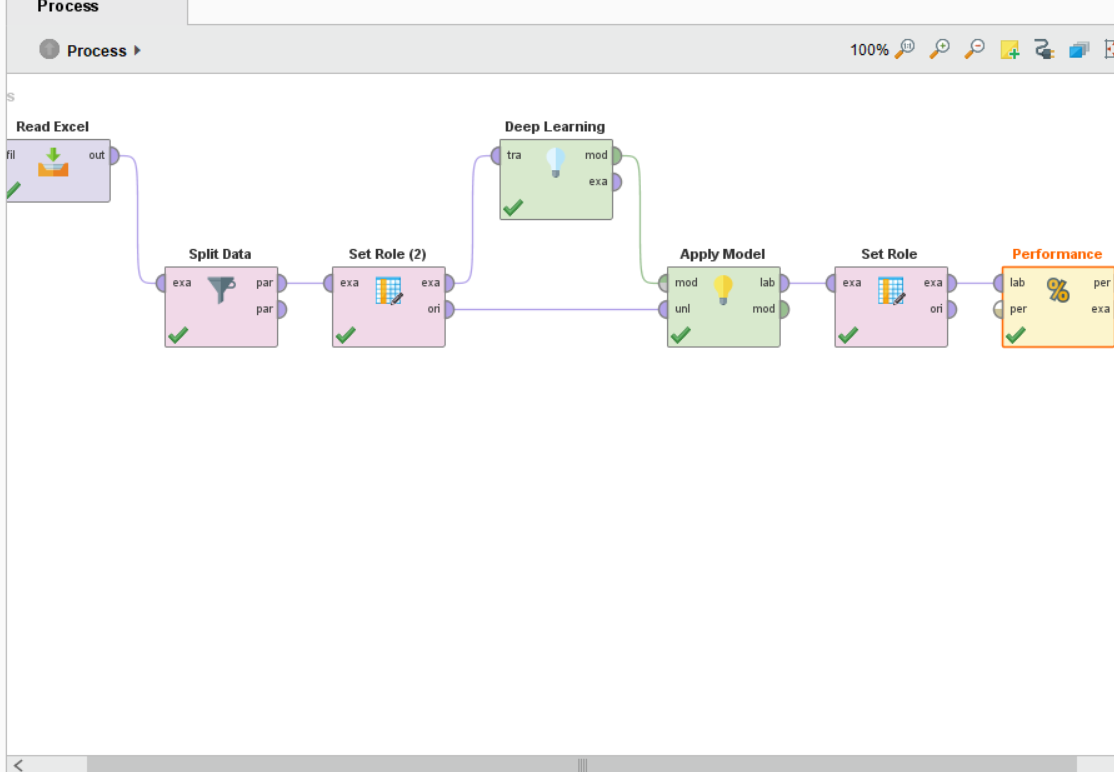# deep learning

Posts: 0Learner III
edited May 2019 in Help
Hi
I want to combine deep learning & neural network in my data because the result of  them are good but when I try to combine them some problems happen. please help me to solve them
Thank you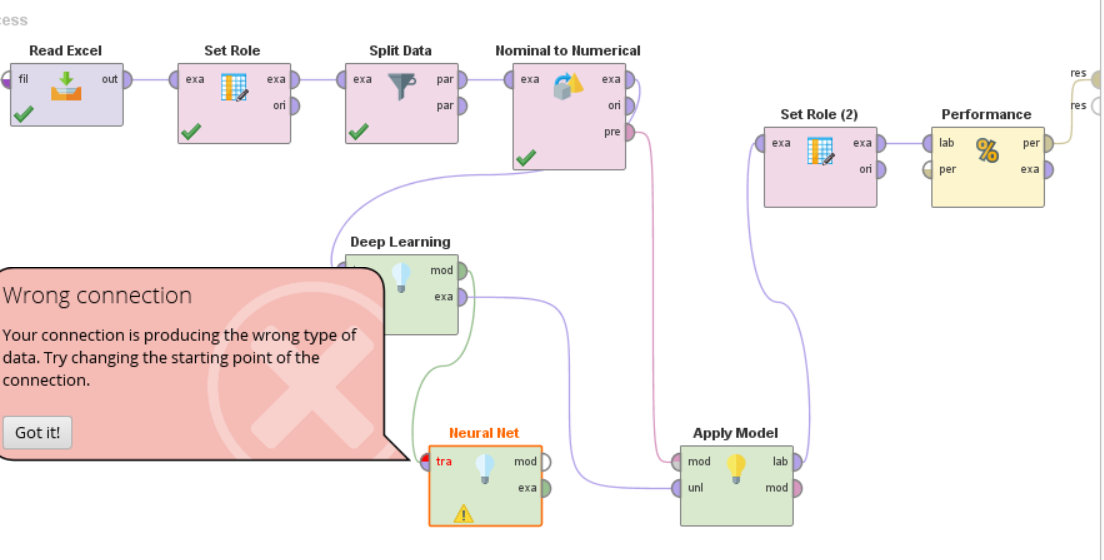Tagged:

• Moderator, Member Posts: 1,207Unicorn
Solution Accepted
@mbs

The simple debugging technique would be to disable all operators after Nominal to numerical operator and connect the output of nominal to numerical operator to results and run your program. Then you can check the statistics of your example set to see if there are any missing values in any attribute of your dataset.

Secondly, adding neural net to a deep learning algorithm is redundant as mentioned by @hughesfleming68, You can check the layer information from neural net operator parameters and add this as a new layer in deep learning operator.
Regards,
Varun
https://www.varunmandalapu.com/

# Be Safe. Follow precautions and Maintain Social Distancing

• Moderator, Member Posts: 1,207Unicorn
Solution Accepted
Then if you want to use the same architecture, I think you can use group models operator and try.
Regards,
Varun
https://www.varunmandalapu.com/

# Be Safe. Follow precautions and Maintain Social Distancing

• Moderator, Member Posts: 1,207Unicorn
edited April 2019 Solution Accepted
It can take multiple models and combine them into a single model. More detailed explanation below.
https://docs.rapidminer.com/8.0/studio/operators/modeling/predictive/group_models.html

When this combined model is applied, it is equivalent to applying the original models in their respective order

Regards,
Varun
https://www.varunmandalapu.com/

# Be Safe. Follow precautions and Maintain Social Distancing

• Moderator, Member Posts: 1,207Unicorn
Solution Accepted
Here is a working example, XML below. Analyze my inputs and outputs carefully and how I am connecting them.

<?xml version="1.0" encoding="UTF-8"?><process version="9.2.001">
<context>
<input/>
<output/>
<macros/>
</context>
<operator activated="true" class="process" compatibility="9.2.001" expanded="true" name="Process">
<parameter key="logverbosity" value="init"/>
<parameter key="random_seed" value="2001"/>
<parameter key="send_mail" value="never"/>
<parameter key="process_duration_for_mail" value="30"/>
<parameter key="encoding" value="SYSTEM"/>
<process expanded="true">
<operator activated="true" class="retrieve" compatibility="9.2.001" expanded="true" height="68" name="Retrieve Titanic Training" width="90" x="45" y="136">
<parameter key="repository_entry" value="//Samples/data/Titanic Training"/>
</operator>
<operator activated="true" class="nominal_to_numerical" compatibility="9.2.001" expanded="true" height="103" name="Nominal to Numerical" width="90" x="179" y="34">
<parameter key="return_preprocessing_model" value="false"/>
<parameter key="create_view" value="false"/>
<parameter key="attribute_filter_type" value="all"/>
<parameter key="attribute" value=""/>
<parameter key="attributes" value=""/>
<parameter key="use_except_expression" value="false"/>
<parameter key="value_type" value="nominal"/>
<parameter key="use_value_type_exception" value="false"/>
<parameter key="except_value_type" value="file_path"/>
<parameter key="block_type" value="single_value"/>
<parameter key="use_block_type_exception" value="false"/>
<parameter key="except_block_type" value="single_value"/>
<parameter key="invert_selection" value="false"/>
<parameter key="include_special_attributes" value="false"/>
<parameter key="coding_type" value="dummy coding"/>
<parameter key="use_comparison_groups" value="false"/>
<list key="comparison_groups"/>
<parameter key="unexpected_value_handling" value="all 0 and warning"/>
<parameter key="use_underscore_in_name" value="false"/>
</operator>
<operator activated="true" class="split_data" compatibility="9.2.001" expanded="true" height="103" name="Split Data" width="90" x="313" y="34">
<enumeration key="partitions">
<parameter key="ratio" value="0.7"/>
<parameter key="ratio" value="0.3"/>
</enumeration>
<parameter key="sampling_type" value="automatic"/>
<parameter key="use_local_random_seed" value="false"/>
<parameter key="local_random_seed" value="1992"/>
</operator>
<operator activated="true" class="multiply" compatibility="9.2.001" expanded="true" height="103" name="Multiply" width="90" x="447" y="136"/>
<operator activated="true" class="h2o:deep_learning" compatibility="9.2.000" expanded="true" height="82" name="Deep Learning" width="90" x="581" y="238">
<parameter key="activation" value="Rectifier"/>
<enumeration key="hidden_layer_sizes">
<parameter key="hidden_layer_sizes" value="50"/>
<parameter key="hidden_layer_sizes" value="50"/>
</enumeration>
<enumeration key="hidden_dropout_ratios"/>
<parameter key="use_local_random_seed" value="false"/>
<parameter key="local_random_seed" value="1992"/>
<parameter key="epochs" value="10.0"/>
<parameter key="compute_variable_importances" value="false"/>
<parameter key="train_samples_per_iteration" value="-2"/>
<parameter key="epsilon" value="1.0E-8"/>
<parameter key="rho" value="0.99"/>
<parameter key="learning_rate" value="0.005"/>
<parameter key="learning_rate_annealing" value="1.0E-6"/>
<parameter key="learning_rate_decay" value="1.0"/>
<parameter key="momentum_start" value="0.0"/>
<parameter key="momentum_ramp" value="1000000.0"/>
<parameter key="momentum_stable" value="0.0"/>
<parameter key="standardize" value="true"/>
<parameter key="L1" value="1.0E-5"/>
<parameter key="L2" value="0.0"/>
<parameter key="max_w2" value="10.0"/>
<parameter key="loss_function" value="Automatic"/>
<parameter key="distribution_function" value="AUTO"/>
<parameter key="early_stopping" value="false"/>
<parameter key="stopping_rounds" value="1"/>
<parameter key="stopping_metric" value="AUTO"/>
<parameter key="stopping_tolerance" value="0.001"/>
<parameter key="missing_values_handling" value="MeanImputation"/>
<parameter key="max_runtime_seconds" value="0"/>
<list key="expert_parameters"/>
<list key="expert_parameters_"/>
</operator>
<operator activated="true" class="neural_net" compatibility="9.2.001" expanded="true" height="82" name="Neural Net" width="90" x="581" y="136">
<list key="hidden_layers"/>
<parameter key="training_cycles" value="200"/>
<parameter key="learning_rate" value="0.01"/>
<parameter key="momentum" value="0.9"/>
<parameter key="decay" value="false"/>
<parameter key="shuffle" value="true"/>
<parameter key="normalize" value="true"/>
<parameter key="error_epsilon" value="1.0E-4"/>
<parameter key="use_local_random_seed" value="false"/>
<parameter key="local_random_seed" value="1992"/>
</operator>
<operator activated="true" class="group_models" compatibility="9.2.001" expanded="true" height="103" name="Group Models" width="90" x="715" y="340"/>
<operator activated="true" class="apply_model" compatibility="9.2.001" expanded="true" height="82" name="Apply Model" width="90" x="715" y="34">
<list key="application_parameters"/>
<parameter key="create_view" value="false"/>
</operator>
<operator activated="true" class="performance_classification" compatibility="9.2.001" expanded="true" height="82" name="Performance" width="90" x="715" y="136">
<parameter key="main_criterion" value="first"/>
<parameter key="accuracy" value="true"/>
<parameter key="classification_error" value="false"/>
<parameter key="kappa" value="false"/>
<parameter key="weighted_mean_recall" value="false"/>
<parameter key="weighted_mean_precision" value="false"/>
<parameter key="spearman_rho" value="false"/>
<parameter key="kendall_tau" value="false"/>
<parameter key="absolute_error" value="false"/>
<parameter key="relative_error" value="false"/>
<parameter key="relative_error_lenient" value="false"/>
<parameter key="relative_error_strict" value="false"/>
<parameter key="normalized_absolute_error" value="false"/>
<parameter key="root_mean_squared_error" value="false"/>
<parameter key="root_relative_squared_error" value="false"/>
<parameter key="squared_error" value="false"/>
<parameter key="correlation" value="false"/>
<parameter key="squared_correlation" value="false"/>
<parameter key="cross-entropy" value="false"/>
<parameter key="margin" value="false"/>
<parameter key="soft_margin_loss" value="false"/>
<parameter key="logistic_loss" value="false"/>
<parameter key="skip_undefined_labels" value="true"/>
<parameter key="use_example_weights" value="true"/>
<list key="class_weights"/>
</operator>
<connect from_op="Retrieve Titanic Training" from_port="output" to_op="Nominal to Numerical" to_port="example set input"/>
<connect from_op="Nominal to Numerical" from_port="example set output" to_op="Split Data" to_port="example set"/>
<connect from_op="Split Data" from_port="partition 1" to_op="Multiply" to_port="input"/>
<connect from_op="Split Data" from_port="partition 2" to_op="Apply Model" to_port="unlabelled data"/>
<connect from_op="Multiply" from_port="output 1" to_op="Neural Net" to_port="training set"/>
<connect from_op="Multiply" from_port="output 2" to_op="Deep Learning" to_port="training set"/>
<connect from_op="Deep Learning" from_port="model" to_op="Group Models" to_port="models in 2"/>
<connect from_op="Neural Net" from_port="model" to_op="Group Models" to_port="models in 1"/>
<connect from_op="Group Models" from_port="model out" to_op="Apply Model" to_port="model"/>
<connect from_op="Apply Model" from_port="labelled data" to_op="Performance" to_port="labelled data"/>
<connect from_op="Performance" from_port="performance" to_port="result 1"/>
<portSpacing port="source_input 1" spacing="0"/>
<portSpacing port="sink_result 1" spacing="0"/>
<portSpacing port="sink_result 2" spacing="0"/>
</process>
</operator>
</process>

Sample screenshot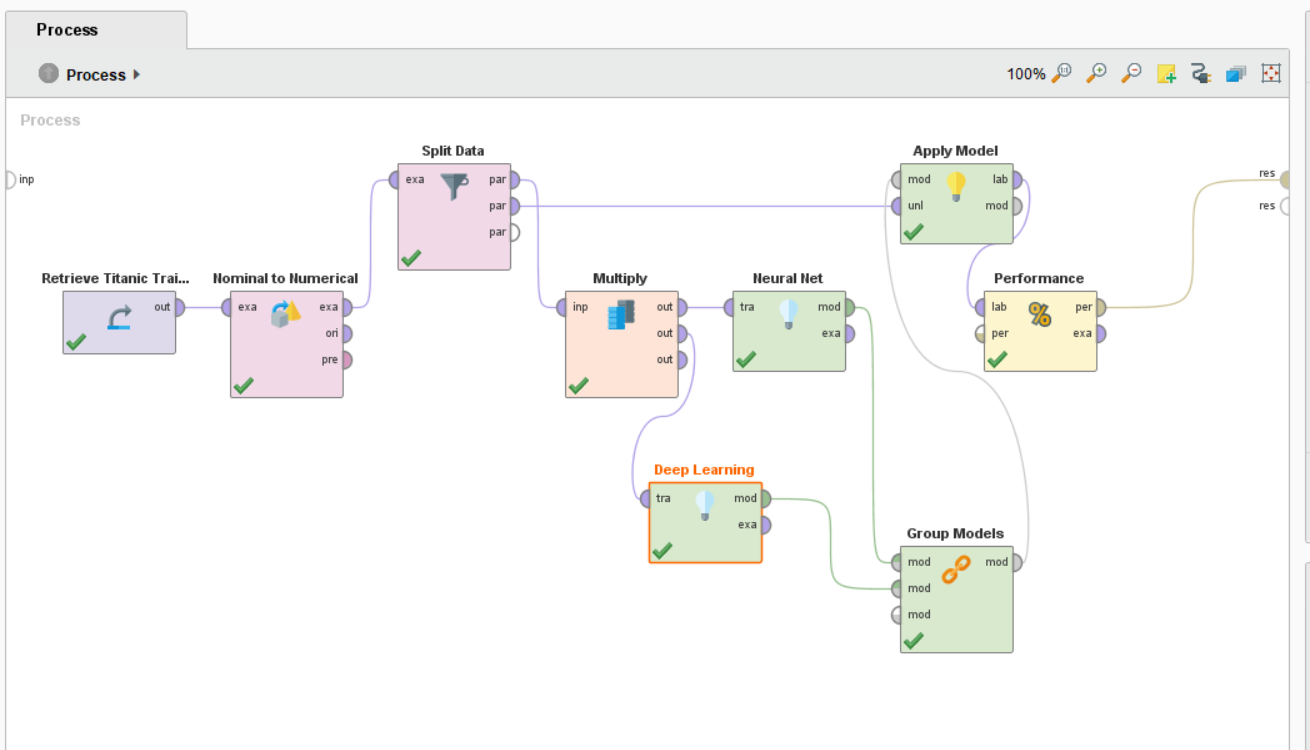Hope this helps.
Regards,
Varun
https://www.varunmandalapu.com/

# Be Safe. Follow precautions and Maintain Social Distancing

• Moderator, Member Posts: 1,207Unicorn
Solution Accepted
Open a new process, copy this code and paste it in XML window (View --> Show Panel --> XML) then click on a green tick mark. you can see the process and run it as well. I am also attaching process for importing but try the XML first so that you will get familiar with ways to use Rapidminer.
Regards,
Varun
https://www.varunmandalapu.com/

# Be Safe. Follow precautions and Maintain Social Distancing

• Moderator, Member Posts: 1,207Unicorn
Solution Accepted
@mbs

Open a new process, copy this code and paste it in XML window (View --> Show Panel --> XML) then click on a green tick mark. you can see the process and run it as well. I am also attaching .rmp process for importing (File --> Import Process) but try the XML first so that you will get familiar with ways to use Rapidminer.
Regards,
Varun
https://www.varunmandalapu.com/

# Be Safe. Follow precautions and Maintain Social Distancing

• Moderator, Member Posts: 1,207Unicorn
edited April 2019 Solution Accepted
Did you check if read excel operator is getting your label column into rapidminer? As I said earlier you can check by disabling all other operators and connecting read excel to output. If there is some issue try importing into RM repository and check. There might be some simple mistake.

@mbs one more thing, you should set role to a label after read excel in case you are splitting data as train and test sets.
Regards,
Varun
https://www.varunmandalapu.com/

# Be Safe. Follow precautions and Maintain Social Distancing

• Moderator, Member Posts: 1,207Unicorn
Solution Accepted
The can be many reasons,

1. How are you splitting data? If it is a random split then "are your setting local random seed parameter" that will give you the same train and test sets all the time.
2. Your algorithm might be overfitting due to a more complex neural net which might be in case of a neural net + deep learning algorithm.
3. Is your dataset balanced (a simlilar number for samples for each output label)? If not, accuracy is not a good performance measure.

For reason  1, I recommend you use cross-validation instead of the random splitting of the train and test datasets. This will give you much reliable results.
For reason 2, you need to start from smaller networks and then build more complex networks based on data and test performance. There is no use of building networks with more hidden layers when a simple neural network can achieve your task.
For reason 3, use AUC and kappa values as performance metrics instead of accuracy.

Regards,
Varun
https://www.varunmandalapu.com/

# Be Safe. Follow precautions and Maintain Social Distancing

• Moderator, Member Posts: 1,207Unicorn
Solution Accepted
For question 1 and reason 1, even if you use split validation, a label which has only one sample can be either in Training or testing dataset, so I don't understand why data with a single sample is in data set.

The reason I am saying this is, your single sample data can either be in training or test dataset. If it is in training, it is never useful in the test dataset to check performance. If it is in test set then it never saw in training which means it will be predicted wrong all the time as its label never existed while the algorithm trained. So I think labels with single samples might not be useful for performance measure. You can use cross-validation with stratified sampling that will try to keep samples related to every class in all subsets.

If you use split validation, you should check "use local random seed" in parameters of split data. This will always create the same train and test subsets even if you use different models.

Reason 2: The complex algorithms overfit some times (depends on data). A deep learning algorithm is the one which has more hidden layers. In my statement, I am saying to train and test a model with a single hidden layer first and then note the performance parameters like accuracy, kappa, etc. Then you can build another model with more hidden layers and see the performances. If your simple model is giving the best performance there is no need to use a complex model with multiple hidden layers.

Reason 3: A kappa value can be between -1 to 1. A positive kappa value between 0 and 1 with higher the better. A negative kappa value between -1 and 0 represent your algorithm is predicting exactly opposite classes for data. For example, if you have 20 samples with 10 labeled as male and 10 labeled as female. A kappa value of zero means, your algorithm is predicting all 20 samples as either male or female. A negative kappa value means, your algorithms are predicting opposite classes, this means male samples are predicted as female and female samples are predicted as male. A positive kappa value means it is trying to predict correct classes for the given samples. Higher kappa means better predictions.

Hope this helps.

Regards,
Varun
https://www.varunmandalapu.com/

# Be Safe. Follow precautions and Maintain Social Distancing

• Moderator, Member Posts: 1,207Unicorn
Solution Accepted
Your process seems correct, instead of split data try using cross-validation. You can use anything but try these things as well so that you can defend your thesis well as you might get questions like "How reliable are your performances?" etc.
Regards,
Varun
https://www.varunmandalapu.com/

# Be Safe. Follow precautions and Maintain Social Distancing

• Moderator, Member Posts: 1,207Unicorn
Solution Accepted
you can remove labels with a single sample from my understanding, I am also not exactly sure on this as this is the first time I am getting a label with a single sample.
Regards,
Varun
https://www.varunmandalapu.com/

# Be Safe. Follow precautions and Maintain Social Distancing

• Moderator, Member Posts: 1,207Unicorn
Solution Accepted
Hello @mbs

Simple mistake, you did not connect "per" port of cross validation to the results. Per means performance
Regards,
Varun
https://www.varunmandalapu.com/

# Be Safe. Follow precautions and Maintain Social Distancing

• Moderator, Member Posts: 1,207Unicorn
Solution Accepted
One last thing,  cross validation results can be lower (bad) than your random split results. The reason is it tests and trains on all data and averages the performance. If there is some bad data, that will reduce the performance. For more details, read about cross validation and you will get to know it. But cross validation will give you reliable results.

Thanks
Regards,
Varun
https://www.varunmandalapu.com/

# Be Safe. Follow precautions and Maintain Social Distancing

• 12Administrator, Moderator, Employee, RapidMiner Certified Analyst, Community Manager, Member, University Professor, PM Moderator Posts: 2,959Community Manager
Solution Accepted
hi @mbs I'd recommend this from the Academy: https://academy.rapidminer.com/learn/video/validating-a-model

You could also try searching the community...there is a rather well-written article on this topic from March 4th.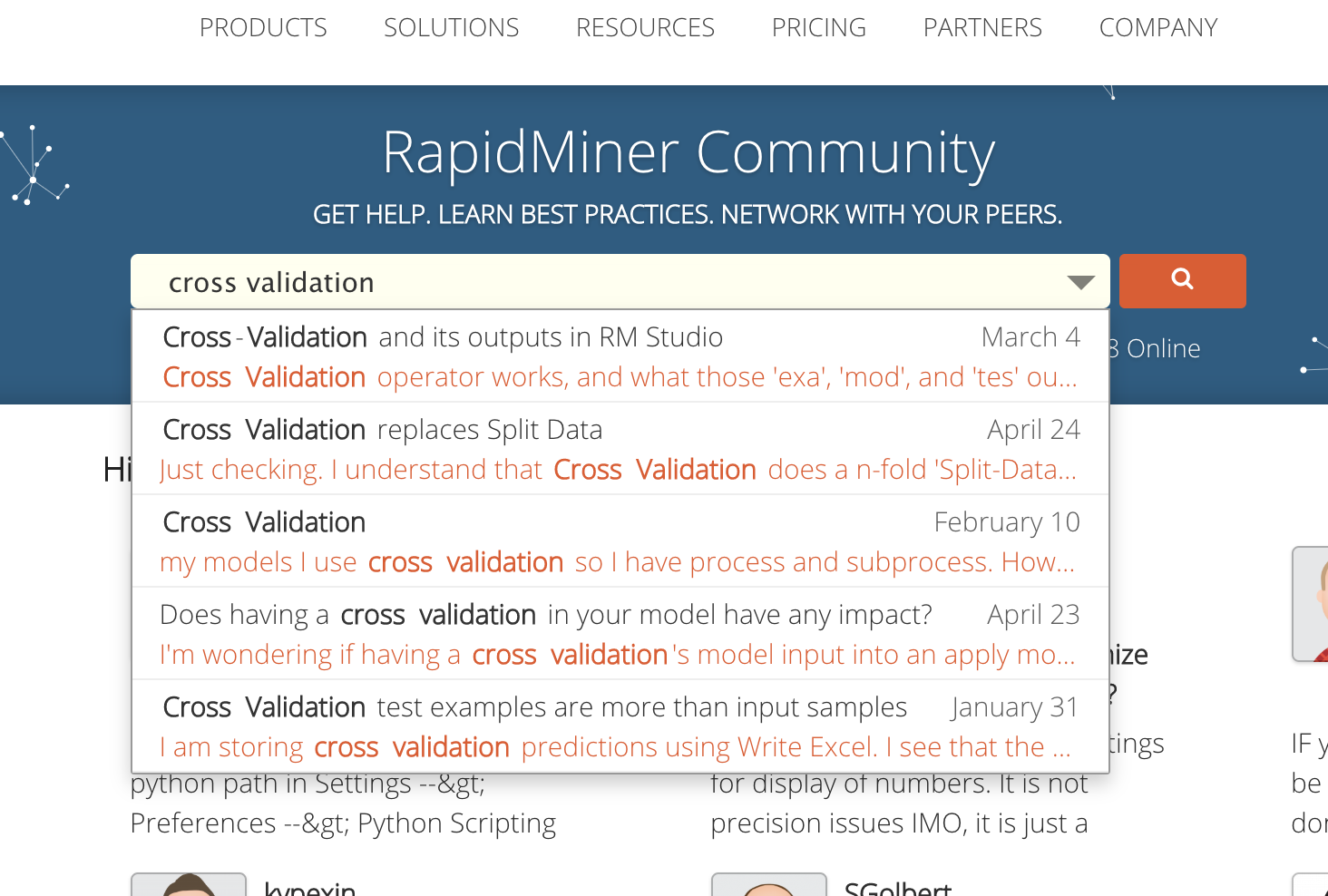• Moderator, Member Posts: 1,207Unicorn
Solution Accepted
Yep, you can select whatever works in your case. If you ask me, 2000 samples is a normal data and I cross-validate data with 100000 samples to get confident results. Again, this might be subjective. Getting good performance and reliable performance are two different things. Try different things and see what is good for your thesis.
Regards,
Varun
https://www.varunmandalapu.com/

# Be Safe. Follow precautions and Maintain Social Distancing

• Moderator, Member Posts: 1,207Unicorn
Solution Accepted
Sorry @mbs, I am swamped working on paper. I can explain it to you. I know you got confused with "smaller network" and "complex network". A neural network can have multiple layers. So, a simple neural network, in my view, is with one hidden layer and a few neurons in it. If you increase the number of hidden layers with a different number of neurons and different activation functions the network is becoming more complex. You can build models with a different number of layers with a neural network operator or deep learning operator or deep learning extension in Rapidminer. I recommend you get a general understanding of neural networks and deep learning (deep neural networks) and try with relevant operators in rapidminer.

If you have any specific question or need clarification I can help in that case but building models take time. I recommend you watch videos and tutorials from rapidminer or any other source that makes you understand easily.
Regards,
Varun
https://www.varunmandalapu.com/

# Be Safe. Follow precautions and Maintain Social Distancing

• Moderator, Member Posts: 1,207Unicorn
Solution Accepted
Hello @mbs

Which models are you trying to combine? I am not sure if there is a way to combine models without group model operator.

Yes, Cross-validation operator will divide your data into multiple subsets that are used for training and testing of algorithms. Yes, testing is done without labels as the trained model is trying to predict the output for a given sample.

Thanks
Regards,
Varun
https://www.varunmandalapu.com/

# Be Safe. Follow precautions and Maintain Social Distancing

• Moderator, Member Posts: 1,207Unicorn
Solution Accepted
Yep, ensemble models work but you should be careful in analyzing higher performance. For this, you need to set aside some data from testing after the model is trained and tested using cross-validation. If the performance of this hold out a dataset is good then your model might be good.

PS: Cross-validation reduces overfitting but complex models tend to overfit even in cross-validation so we should be careful in analyzing very good results.
Regards,
Varun
https://www.varunmandalapu.com/

# Be Safe. Follow precautions and Maintain Social Distancing

• Moderator, Member Posts: 1,207Unicorn
Solution Accepted
Sorry, don't get confused. What I am saying is a validation process we do when we observe high performances like 99 percent accuracy etc., We split the dataset into 0.8 to 0.2 ratio and cross-validate the 0.8 portions of the dataset and then we connect the model output of cross-validation operator to test the 0.2 percent dataset. Now we can have a performance from cross-validation and hold out (0.2) dataset as well.

If you think this is confusing you can go with your current results.
Regards,
Varun
https://www.varunmandalapu.com/

# Be Safe. Follow precautions and Maintain Social Distancing

• Moderator, Member Posts: 1,207Unicorn
Solution Accepted
Dear friend, here is the famous Titanic dataset used for cross-validation and verification of results. You can change the process as you like.

Please be more specific about graphs. Which graphs are you talking about?

Also, this thread has become so long I guess.
Regards,
Varun
https://www.varunmandalapu.com/

# Be Safe. Follow precautions and Maintain Social Distancing

• Moderator, Member Posts: 1,207Unicorn
Solution Accepted
Hello @mbs

There are two performances you can look from my process. One is related to cross-validation performance and the other is related to Hold-out data set performance. Can you inform these two performances (both accuracies and kappa's)?
Regards,
Varun
https://www.varunmandalapu.com/

# Be Safe. Follow precautions and Maintain Social Distancing

• Moderator, Member Posts: 1,207Unicorn
Solution Accepted
They are separate. The cross-validation operator I used in the process will give accuracy and kappa values as this is applied to 80% of data. Then you have a test data (I named as hold out data) of 20% which is used to test the model if it is showing consistent performance or not, you will have performances for this 20 % data as well (I named that performance operator as hold-out performance in your process).
Regards,
Varun
https://www.varunmandalapu.com/

# Be Safe. Follow precautions and Maintain Social Distancing

«13

• Posts: 0Learner III
next try and next problem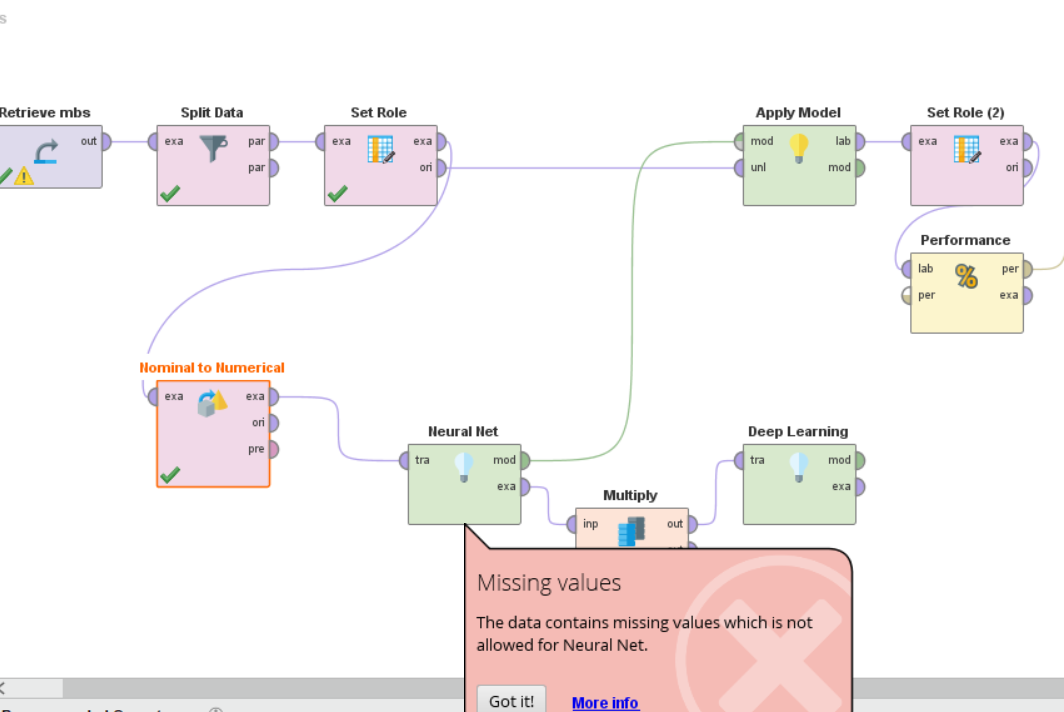• Member Posts: 323Unicorn
In your first example, you need to review ensembles. Go through the examples but combining a neural net with a deep learner is somewhat redundant. You might consider just adding extra layers to your deep learner.

In your second example, you need to eliminate or interpolate your missing examples so that you don't have any gaps in your data.
• Posts: 0Learner III
hughesfleming68
But would you please explain more about extra layer? I can not understand.
In my second example I dont have any missing values but the result of Rm is not clear.I mean that "MISSING VALUE" doesnt have meaning in this situation.
Thanks• Posts: 0Learner III
HI @varunm1
Thank you for your suggestionI think this is very complex and I have to try it moreI have to work on your suggestion because I can not understand it completely.• Posts: 0Learner III
@varunm1
Thank you for your perfect ideawould you please explain group model more?• Posts: 0Learner IIIperfect

• Posts: 0Learner III
@varunm1
please look at the problem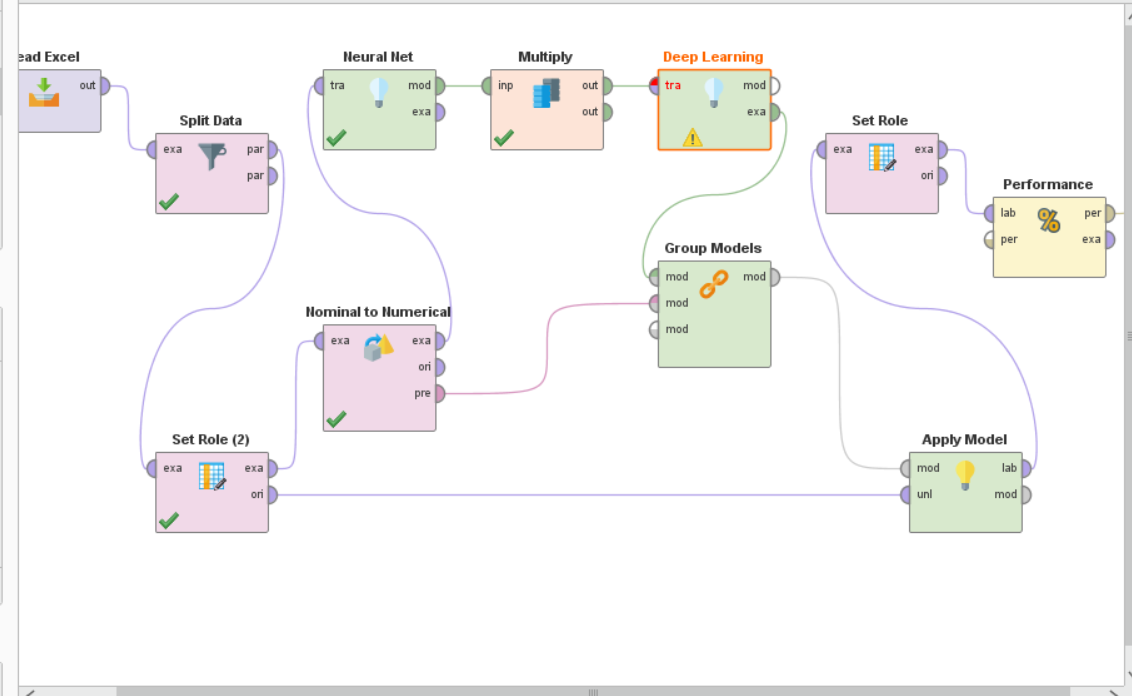• Posts: 0Learner III
edited April 2019
varunm1

This is xml code.
how can I import it to my RM to see the result?• Posts: 0Learner III
I see the screenshot now.
perfect great• Posts: 0Learner III
edited April 2019
varunm1
mine doesnt workit needs alot of extra operator
how can I import your xml code?
• Posts: 0Learner III
yes I will do that• Posts: 0Learner III
varunm1
I coped your xml and I changed the data but I dont know why again I miss my last column of my data which is my labelAny way @ varunm1  you and your group in Rapidminer are  brilliant.
• Posts: 0Learner III
edited April 2019
@varunm1
I will check all the points that you mention again but Unfortunately this is a bug in RM that some of my friend and me talked about that before. if you look at this link you will understand the problem.• Posts: 0Learner III
edited April 2019
@varunm1
finally I solve the problem. According to the link that I send before I copy the excel and put it in a new folder and rename it then use read excel and recall data from my desktop and it works.But I did it from your xml code and just I changed the data.
Thanks• Posts: 0Learner III
The accuracy of that is 95% but when I use neural network or deep learning the accuracy of each one was 99.65%
@varunm1 do you know why the result of  combination is not as good as the first single try of them?
• Posts: 0Learner III
edited April 2019
question1: 0.7 and 0.3 with split dat.
question2:yes youre right.
question3: no, it's not.
reason1: cross validation doesnt work in my RM. just a point: I have just one sample in some of my label so I think cross validation is not good.
reason2: sorry I can not understand. please explain it moer.
reason3: Iheard and read some paper for AUC but it was not clear and also I dont have any information about kappa. so please explain them.
varunm1
thank you for the time that you spend on my question• Posts: 0Learner III
edited April 2019
varunm1
your explanation was perfect.
This is my thesis and I downloaded around 2000 data for that and it was unsupervised then I put label for that and made it supervised. at first I had to do datamining for that but then I change it to machine  learning.
The point is that I recall all the labeled data at once in to RM and just with split data divided it. Is it correct?
Thank you
Regards
mbs

• Posts: 0Learner III
edited April 2019
With kappa the result is 0.995
and also I try to use different algorithms or combine them in order to compare the result
if you think one data in some labels are wrong I will delete them
• Posts: 0Learner III
please look at the process